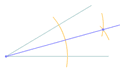# Bisect

"Bisect" means to divide into two equal parts.

You can bisect lines, angles, and more.

The dividing line is called the "bisector"

## Bisecting a Line Segment

Here the blue line segment is bisected by the red line:You can try it yourself (try moving the points):

When the bisector is perpendicular (at right angles) to the line being bisected it is called a "perpendicular bisector".

## Bisecting an Angle

Here the blue angle is bisected by the red line:You can try it yourself (try moving the points):

images/geom-angle-bisect.js## Bisect a Shape

We can also bisect some shapes. Here a kite is bisected by a dashed line: